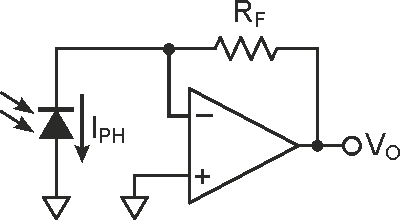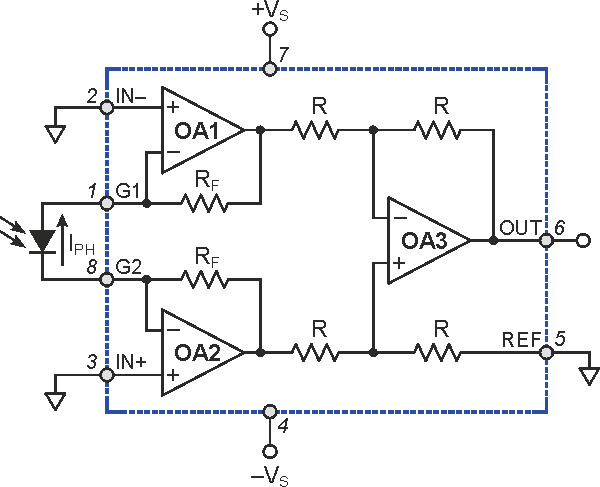# Instrumentation amp makes an accurate transimpedance amp too

## Texas Instruments INA129 PGA204

Stefano Salvatori

EDN

Transimpedance amplifier circuits are often used to convert photodiode current to a voltage signal. In the conventional transimpedance amplifier schematic of Figure 1, the current-to-voltage conversion factor is given by the value of the op-amp feedback resistor RF. Obviously, neglecting any non-ideal behavior of the op-amp, the accuracy of the I-V converter is tied to that of RF (on both its absolute value and thermal coefficient).Figure 1. A classical transimpedance amplifier for photodiode signal conditioning. The current-to-voltage conversion factor is given by RF.

High precision thin film resistors are available, either as a single components or as an array of matched-value resistors. In both cases, typical tolerance of commercially available precision resistors is ±0.1%, and ratio tolerances are commonly found in the 0.02% - 0.05% range. The cost of such components may dominate, being easily greater than that of a low-noise, low-offset, precision op-amp. This Design Idea presents an alternate approach.

Monolithic three- op-amp instrumentation amplifiers (IAs) usually integrate laser-trimmed precision resistors, with accurate absolute values for the first-stage feedback parts, and high matching-accuracy for those around the output difference amplifier (see, for example, the AD620 or INA129). The overall gain error and gain non-linearity can be as low as ±0.01% and ±0.001%, respectively. Also thermal coefficient is very low – on the order of tens of ppm/K.Figure 2. An instrumentation amplifier integrates precision matched resistors. Feedback resistors typically have absolute tolerances as low as ±0.01%. This configuration turns the IA into a transimpedance amplifier for photodiode signal conditioning with transimpedance equal to 2 × RF.

Programmable IA integrated circuits usually have a design as in Figure 2. The connections shown allow one to realize a highly accurate current-to-voltage converter having a transimpedance dependent only on the RF value of the IA input stage. Indeed, neglecting OA1 and OA2 inverting-input bias currents, the same photodiode IPH current flows in the two RF feedback resistors. Since the IN(+) and IN(-) input pins are connected to ground, the OA3 difference amplifier will see RF × IPH and -RF × IPH voltages at its inputs. The IA output voltage VO is:

VO = 2 × RF × IPH                  (1)

where IPH is the photodiode photocurrent. The output, VO, will be positive, as the IPH current flows in the direction shown in Figure 2.

If a bias voltage is necessary for photodetector biasing (a reverse bias for a photodiode, or a polarization for a photoresistor), the component can be connected with opposite polarity at the G1/G2 pins compared to that in Figure 2. Then, VBIAS can be applied to the IN(-) input of OA1 such that the same potential is virtually maintained by OA1 at the detector cathode. If the same voltage is applied to the IA’s REF input, Equation (1) still remains valid, though watch the allowed common-mode voltage amplitude on the IA inputs.

A typical circuit’s VO will range from a few millivolts to a few volts; fixed gain, but adequate for many applications. In order to overcome this limitation, a precision programmable-gain amplifier (e.g., the PGA204) can follow the circuit of Figure 2.

## Materials on the topic

EDN